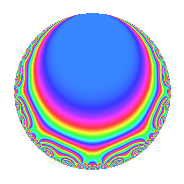# Properties

 Label 4004.2.a.dLevel 4004 Weight 2 Character orbit 4004.a Self dual yes Analytic conductor 31.972 Analytic rank 1 Dimension 4 CM no Inner twists 1

# Related objects

## Newspace parameters

 Level: $$N$$ = $$4004 = 2^{2} \cdot 7 \cdot 11 \cdot 13$$ Weight: $$k$$ = $$2$$ Character orbit: $$[\chi]$$ = 4004.a (trivial)

## Newform invariants

 Self dual: yes Analytic conductor: $$31.9721009693$$ Analytic rank: $$1$$ Dimension: $$4$$ Coefficient field: 4.4.3981.1 Coefficient ring: $$\Z[a_1, a_2, a_3]$$ Coefficient ring index: $$1$$ Twist minimal: yes Fricke sign: $$1$$ Sato-Tate group: $\mathrm{SU}(2)$

## $q$-expansion

Coefficients of the $$q$$-expansion are expressed in terms of a basis $$1,\beta_1,\beta_2,\beta_3$$ for the coefficient ring described below. We also show the integral $$q$$-expansion of the trace form.

 $$f(q)$$ $$=$$ $$q + ( -1 - \beta_{3} ) q^{3} + ( \beta_{2} + \beta_{3} ) q^{5} + q^{7} + ( 1 - \beta_{1} + 2 \beta_{3} ) q^{9} +O(q^{10})$$ $$q + ( -1 - \beta_{3} ) q^{3} + ( \beta_{2} + \beta_{3} ) q^{5} + q^{7} + ( 1 - \beta_{1} + 2 \beta_{3} ) q^{9} + q^{11} + q^{13} + ( -1 - \beta_{1} ) q^{15} + ( -2 - \beta_{2} - \beta_{3} ) q^{17} + ( -2 - \beta_{1} - \beta_{2} ) q^{19} + ( -1 - \beta_{3} ) q^{21} + ( 3 \beta_{1} - \beta_{2} ) q^{23} + ( -2 + 2 \beta_{1} - 2 \beta_{2} - 3 \beta_{3} ) q^{25} + ( -3 + 3 \beta_{1} + \beta_{2} ) q^{27} + ( -2 + 3 \beta_{1} - 2 \beta_{3} ) q^{29} + ( -1 + 2 \beta_{1} - 2 \beta_{3} ) q^{31} + ( -1 - \beta_{3} ) q^{33} + ( \beta_{2} + \beta_{3} ) q^{35} + ( -3 - 3 \beta_{1} + 3 \beta_{3} ) q^{37} + ( -1 - \beta_{3} ) q^{39} + ( -3 - 2 \beta_{1} - 3 \beta_{2} ) q^{41} + ( 1 - 3 \beta_{1} - 2 \beta_{2} + 2 \beta_{3} ) q^{43} + ( 2 + \beta_{1} - 2 \beta_{2} - 2 \beta_{3} ) q^{45} + ( -3 \beta_{1} - 3 \beta_{2} ) q^{47} + q^{49} + ( 3 + \beta_{1} + 2 \beta_{3} ) q^{51} + ( \beta_{1} + \beta_{2} - 3 \beta_{3} ) q^{53} + ( \beta_{2} + \beta_{3} ) q^{55} + ( 1 + 3 \beta_{1} + \beta_{2} + \beta_{3} ) q^{57} + ( -1 - \beta_{1} + 2 \beta_{2} + 2 \beta_{3} ) q^{59} + ( -4 + 3 \beta_{1} + 4 \beta_{2} + \beta_{3} ) q^{61} + ( 1 - \beta_{1} + 2 \beta_{3} ) q^{63} + ( \beta_{2} + \beta_{3} ) q^{65} + ( 1 - 2 \beta_{1} + 2 \beta_{2} + 2 \beta_{3} ) q^{67} + ( -5 - \beta_{1} - 3 \beta_{2} - \beta_{3} ) q^{69} + ( 2 - 4 \beta_{1} + 4 \beta_{3} ) q^{71} + ( -4 + 7 \beta_{1} + 2 \beta_{2} - 3 \beta_{3} ) q^{73} + ( 5 - \beta_{1} - 2 \beta_{2} + 3 \beta_{3} ) q^{75} + q^{77} + ( 3 - \beta_{2} + 5 \beta_{3} ) q^{79} + ( -1 - 2 \beta_{1} - 3 \beta_{2} - 2 \beta_{3} ) q^{81} + ( 4 - 7 \beta_{1} + \beta_{3} ) q^{83} + ( -3 - 2 \beta_{1} + \beta_{3} ) q^{85} + ( 5 - 5 \beta_{1} - 3 \beta_{2} + 4 \beta_{3} ) q^{87} + ( 4 - 5 \beta_{1} + 3 \beta_{2} + 2 \beta_{3} ) q^{89} + q^{91} + ( 5 - 4 \beta_{1} - 2 \beta_{2} + 3 \beta_{3} ) q^{93} + ( -2 - 2 \beta_{1} - 2 \beta_{2} - \beta_{3} ) q^{95} + ( -4 - 2 \beta_{1} - \beta_{2} - 2 \beta_{3} ) q^{97} + ( 1 - \beta_{1} + 2 \beta_{3} ) q^{99} +O(q^{100})$$ $$\operatorname{Tr}(f)(q)$$ $$=$$ $$4q - 3q^{3} - q^{5} + 4q^{7} + q^{9} + O(q^{10})$$ $$4q - 3q^{3} - q^{5} + 4q^{7} + q^{9} + 4q^{11} + 4q^{13} - 5q^{15} - 7q^{17} - 9q^{19} - 3q^{21} + 3q^{23} - 3q^{25} - 9q^{27} - 3q^{29} - 3q^{33} - q^{35} - 18q^{37} - 3q^{39} - 14q^{41} - q^{43} + 11q^{45} - 3q^{47} + 4q^{49} + 11q^{51} + 4q^{53} - q^{55} + 6q^{57} - 7q^{59} - 14q^{61} + q^{63} - q^{65} - 20q^{69} - 6q^{73} + 16q^{75} + 4q^{77} + 7q^{79} - 4q^{81} + 8q^{83} - 15q^{85} + 11q^{87} + 9q^{89} + 4q^{91} + 13q^{93} - 9q^{95} - 16q^{97} + q^{99} + O(q^{100})$$

Basis of coefficient ring in terms of a root $$\nu$$ of $$x^{4} - x^{3} - 4 x^{2} + 2 x + 1$$:

 $$\beta_{0}$$ $$=$$ $$1$$ $$\beta_{1}$$ $$=$$ $$\nu$$ $$\beta_{2}$$ $$=$$ $$\nu^{2} - \nu - 2$$ $$\beta_{3}$$ $$=$$ $$\nu^{3} - \nu^{2} - 3 \nu + 1$$
 $$1$$ $$=$$ $$\beta_0$$ $$\nu$$ $$=$$ $$\beta_{1}$$ $$\nu^{2}$$ $$=$$ $$\beta_{2} + \beta_{1} + 2$$ $$\nu^{3}$$ $$=$$ $$\beta_{3} + \beta_{2} + 4 \beta_{1} + 1$$

## Embeddings

For each embedding $$\iota_m$$ of the coefficient field, the values $$\iota_m(a_n)$$ are shown below.

For more information on an embedded modular form you can click on its label.

Label $$\iota_m(\nu)$$ $$a_{2}$$ $$a_{3}$$ $$a_{4}$$ $$a_{5}$$ $$a_{6}$$ $$a_{7}$$ $$a_{8}$$ $$a_{9}$$ $$a_{10}$$
1.1
 −0.318459 2.28400 0.785261 −1.75080
0 −2.82166 0 0.241539 0 1.00000 0 4.96179 0
1.2 0 −1.84617 0 1.77882 0 1.00000 0 0.408340 0
1.3 0 0.488200 0 −3.65683 0 1.00000 0 −2.76166 0
1.4 0 1.17963 0 0.636469 0 1.00000 0 −1.60847 0
 $$n$$: e.g. 2-40 or 990-1000 Significant digits: Format: Complex embeddings Normalized embeddings Satake parameters Satake angles

## Inner twists

This newform does not admit any (nontrivial) inner twists.

## Twists

By twisting character orbit
Char Parity Ord Mult Type Twist Min Dim
1.a even 1 1 trivial 4004.2.a.d 4

By twisted newform orbit
Twist Min Dim Char Parity Ord Mult Type
4004.2.a.d 4 1.a even 1 1 trivial

## Atkin-Lehner signs

$$p$$ Sign
$$2$$ $$-1$$
$$7$$ $$-1$$
$$11$$ $$-1$$
$$13$$ $$-1$$

## Hecke kernels

This newform subspace can be constructed as the kernel of the linear operator $$T_{3}^{4} + 3 T_{3}^{3} - 2 T_{3}^{2} - 6 T_{3} + 3$$ acting on $$S_{2}^{\mathrm{new}}(\Gamma_0(4004))$$.